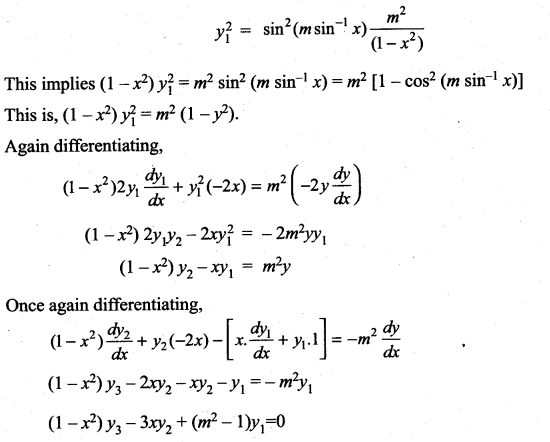# Samacheer Kalvi 11th Maths Solutions Chapter 10 Differentiability and Methods of Differentiation Ex 10.4

## Tamilnadu Samacheer Kalvi 11th Maths Solutions Chapter 10 Differentiability and Methods of Differentiation Ex 10.4

Find the derivatives of the following functions
Question 1.
y = xcos x
Solution:
y = xcos x
Taking log on both sides
log y = log xcos x = cos x log x
differentiating w.r.to x we get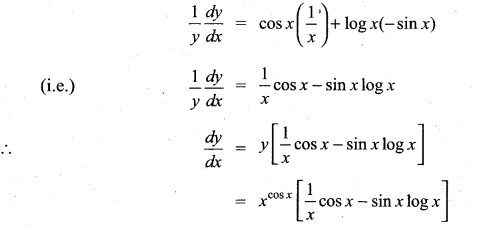Question 2.
y = xlogx + (logx)x
Solution:
y = xlogx + (logx)x
Let y = u + v
Then $$\frac{d y}{d x}=\frac{d u}{d x}+\frac{d v}{d x}$$
u = xlogx
Taking log on both sides
log u = log x log x = log (x)2
differentiating w.r.to x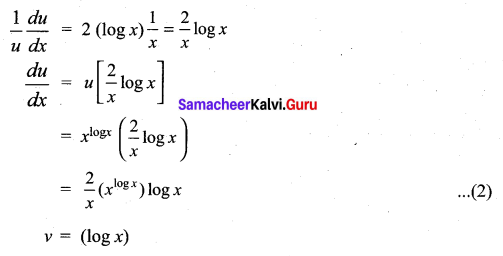Taking log on both sides
log u = log (logx)x = x log (log x)
differentiating w.r.to x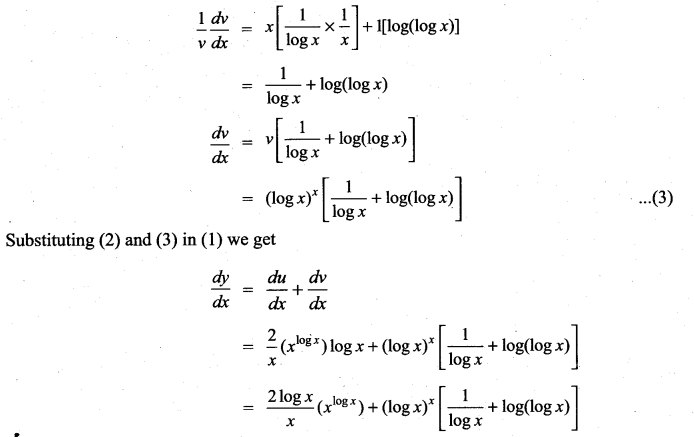Question 3.
$$\sqrt{x y}$$ = e(x – y)
Solution: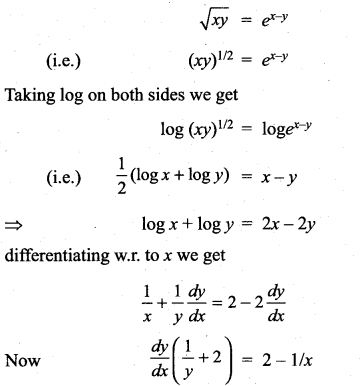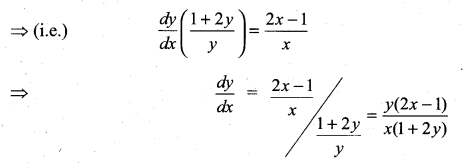Question 4.
xy = yx
Solution:
xy = yx
Taking log on both sides
logxy = logyx
(i.e.) y log x = x log y
differentiating w.r.to x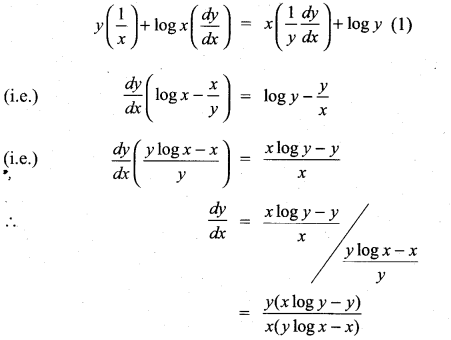Question 5.
(cos x)log x
Solution:
y = (cos x)log x
Taking log on both sides
log y = log (cos x)log x = log x (log cos x)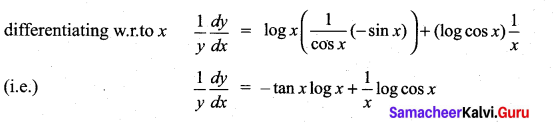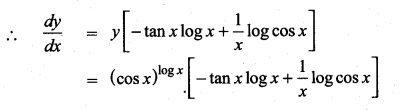Question 6.
$$\frac{x^{2}}{a^{2}}+\frac{y^{2}}{b^{2}}$$ = 1
Solution:
$$\frac{x^{2}}{a^{2}}+\frac{y^{2}}{b^{2}}$$ = 1
Differentiating w.r.to x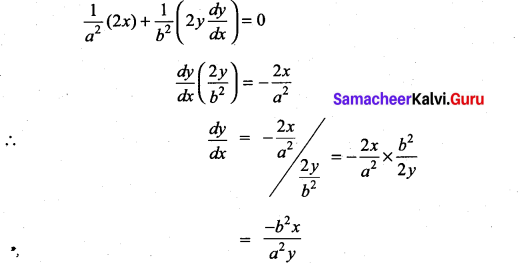Question 7.
$$\sqrt{x^{2}+y^{2}}=\tan ^{-1}\left(\frac{y}{x}\right)$$
Solution:
$$\sqrt{x^{2}+y^{2}}=\tan ^{-1}\left(\frac{y}{x}\right)$$
Differentiating w.r.to x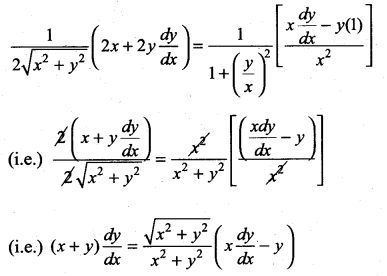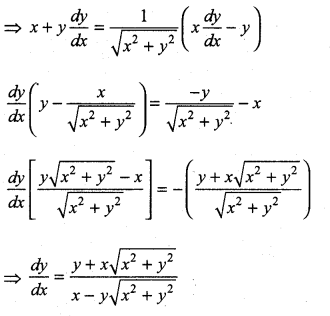Question 8.
tan (x + y) + tan (x – y) = x
Solution:
tan (x + y) + tan (x – y) = x
Differentiating w.r.to x we get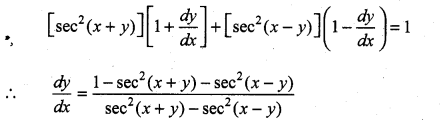Question 9.
If cos (xy) = x, show that $$\frac{d y}{d x}=\frac{-(1+y \sin (x y))}{x \sin x y}$$
Solution:
cos (xy) = x
Differentiating w.r.to x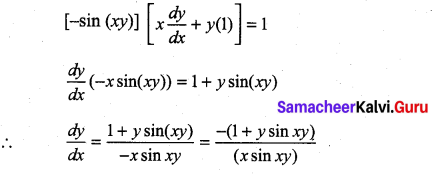Question 10.
$$\tan ^{-1} \sqrt{\frac{1-\cos x}{1+\cos x}}$$
Solution: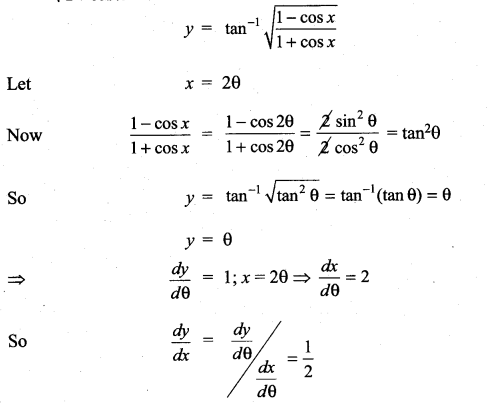Question 11.
$$\tan ^{-1}\left(\frac{6 x}{1-9 x^{2}}\right)$$
Solution: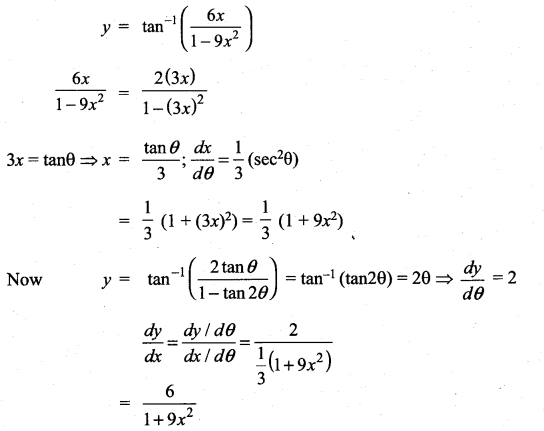Question 12.
cos [2 $$\tan ^{-1} \sqrt{\frac{1-x}{1+x}}$$]
Solution: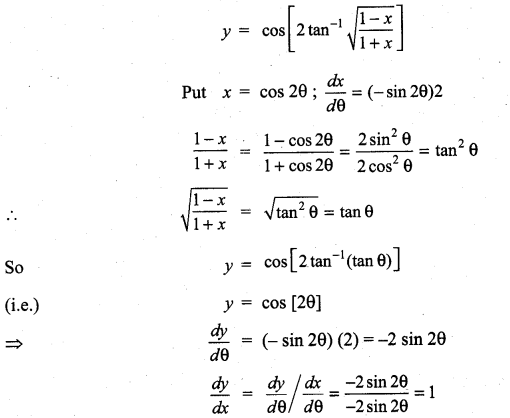Question 13.
x = a cos3t; y = a sin2t
Solution: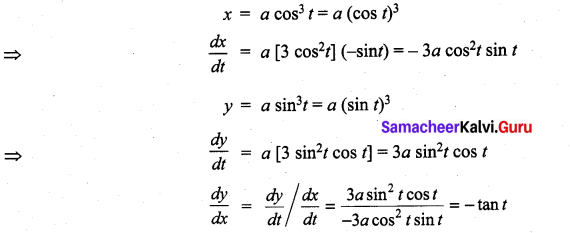Question 14.
x = a (cos t + t sin t); y = a [sin t – t cos t]
Solution: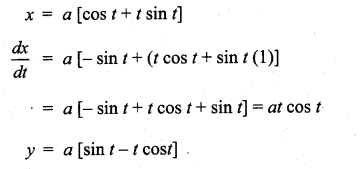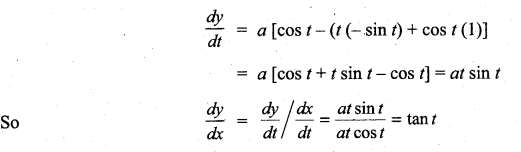Question 15.
x = $$\frac{1-t^{2}}{1+t^{2}}$$; y = $$\frac{2 t}{1+t^{2}}$$
Solution: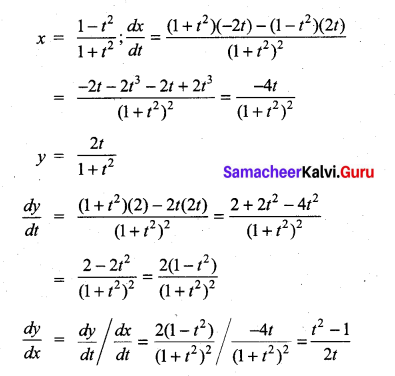Question 16.
$$\cos ^{-1}\left(\frac{1-x^{2}}{1+x^{2}}\right)$$
Solution: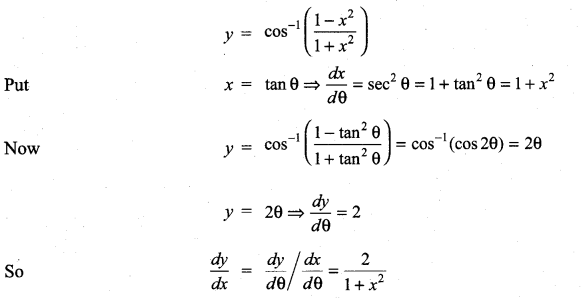Question 17.
sin-1 (3x – 4x3)
Solution: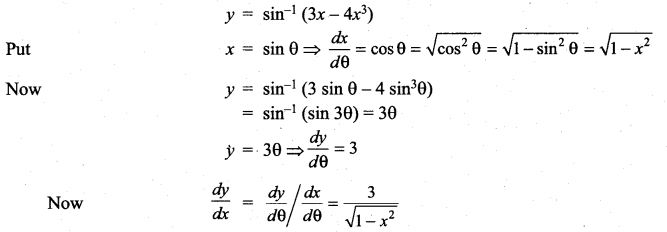Question 18.
$$\tan ^{-1}\left(\frac{\cos x+\sin x}{\cos x-\sin x}\right)$$
Solution: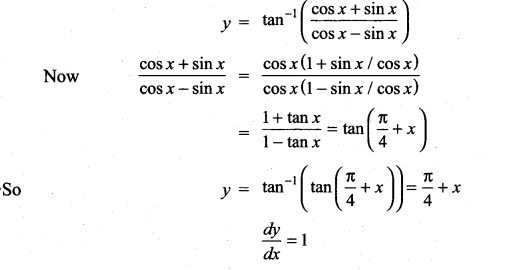Question 19.
Find the derivative of sin x2 with respect to x2
Solution: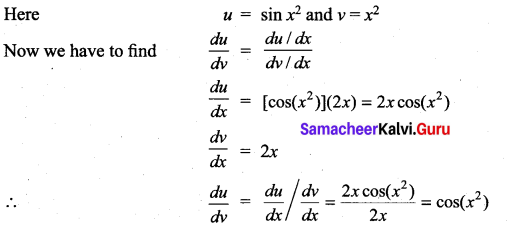Question 20.
Find the derivative of $$\sin ^{-1}\left(\frac{2 x}{1+x^{2}}\right)$$ with respect to tan-1 x.
Solution:
Let u = $$\sin ^{-1}\left(\frac{2 x}{1+x^{2}}\right)$$ and v = tan-1 x
Now we have to find $$\frac{d u}{d v}$$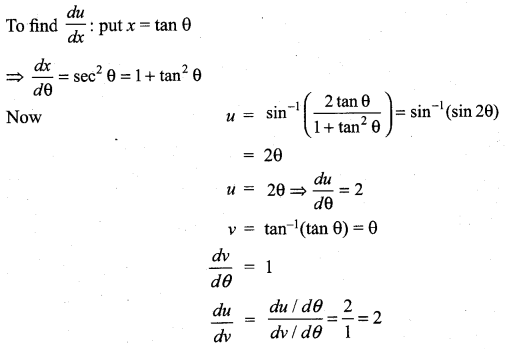Question 21.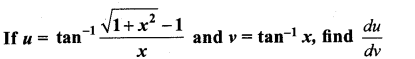Solution: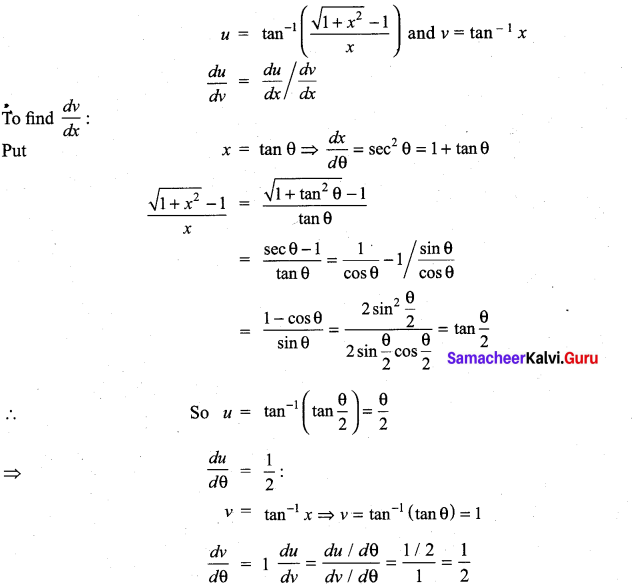Question 22.
Find the derivative with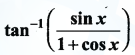with respect to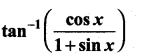Solution: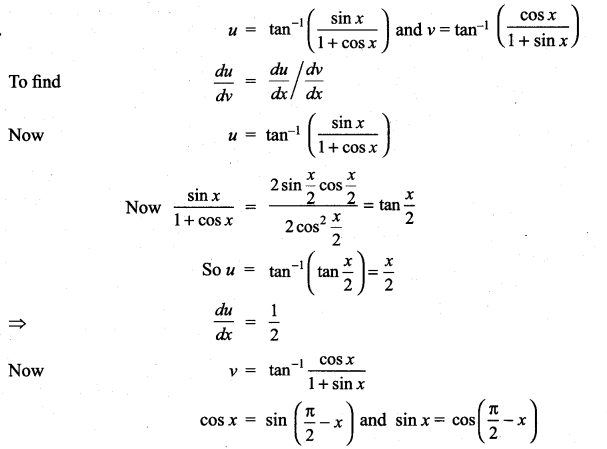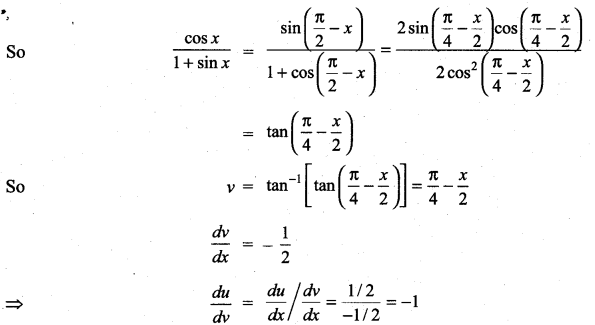Question 23.
If y = sin-1 then find y”.
Solution: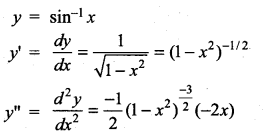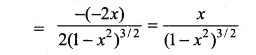Question 24.
If y = etan-1x, show that (1 + x2) y” + (2x – 1) y’ = 0
Solution:
y = etan-1x
y = etan-1x $$\left(\frac{1}{1+x^{2}}\right)$$
⇒ y’ = $$\frac{y}{1+x^{2}}$$ ⇒ y'(1 + x2) = y
differentiating w.r.to x
y’ (2x) + (1 + x2) (y”) = y’
(i.e.) (1 + x2) y” + y’ (2x) – y’ = 0
(i.e.) (1 + x2) y” + (2x – 1) y’ = 0

Question 25.
If y = $$\frac{\sin ^{-1} x}{\sqrt{1-x^{2}}}$$ show that (1 – x2) y2 – 3xy1 – y = 0
Solution: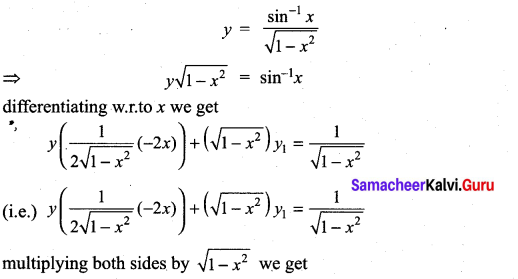-xy + (1 – x2) y1 = 1
differentiating both sides again w.r.to x
-[x y1 + y (1)] + (1 – x2) (y2) + y1 (-2x) = 0
(i.e.) -xy1 – y + (1 – x2) y2 – 2xy1 = 0
(1 – x2) y2 – 3xy1 – y = 0

Question 26.
If x = a (θ + sin θ), y = a (1 – cos θ) then prove that at θ = $$\frac{\pi}{2}$$, y” = $$\frac{1}{a}$$
Solution: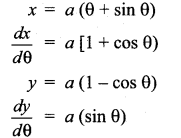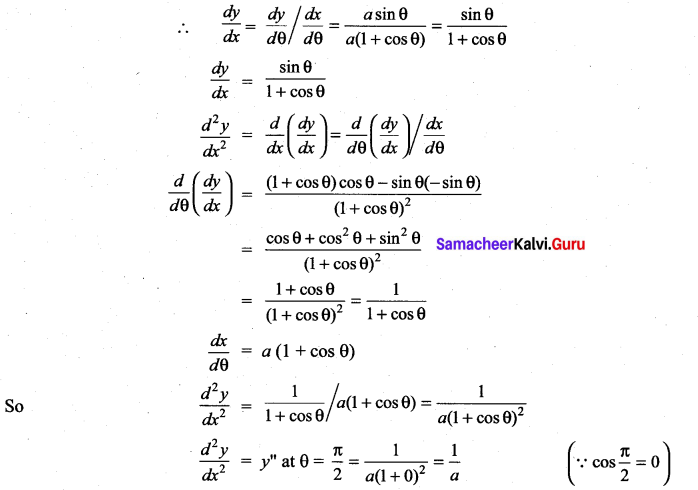Question 27.
If sin y = x sin (a + y) Then prove that $$\frac{d y}{d x}=\frac{\sin ^{2}(a+y)}{\sin a}$$, a ≠ nπ
Solution: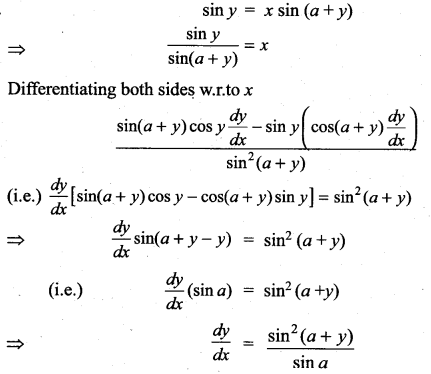Question 28.
If y = (cos-1 x)2, prove that (1 – x2) $$\frac{d^{2} y}{d x^{2}}-x \frac{d y}{d x}$$ – 2 = 0. Hence find y2 when x = 0.
Solution: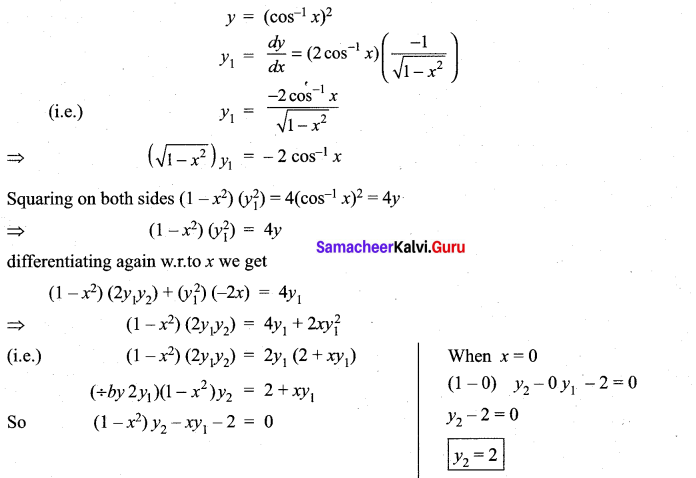### Samacheer Kalvi 11th Maths Solutions Chapter 10 Differentiability and Methods of Differentiation Ex 10.4 Additional Problems

Question 1.
If y = A cos4x + B sin 4x, A and B are constants then Show that y2 + 16y = 0
Solution: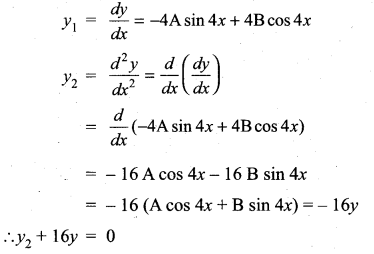Question 2.
If y = cos (m sin-1 x), prove that (1 – x2) y3 – 3xy2 + (m2 – 1) y1 = 0
Solution:
We have y = cos (m sin-1 x)
y1 = sin (m sin-1x). $$\frac{m}{\sqrt{1-x^{2}}}$$